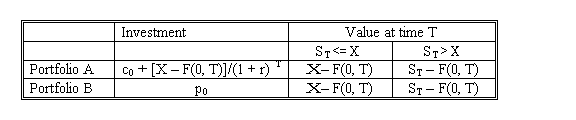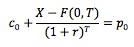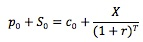### Why should I choose AnalystNotes?

AnalystNotes specializes in helping candidates pass. Period.

##### Subject 2. Put-Call-Forward Parity
Assume that:

• F(0, T): the price established today for a forward contract expiring at time T
• c0: the call option price today
• p0: the put option price today
• Both options expire when the forward contract expires: the time until expiration is also T.
• The exercise price of both options is X.

Consider two portfolios. Portfolio A consists of a long call and a long position in a zero-coupon bond with face value of X - F(0, T). Portfolio B consists of a long put and a long forward.As the two portfolios have exactly the same payoff, their initial investments should be the same as well. That is:This equation is put-call parity for options on forward contracts.

As F(0, T) = S0(1 + r)T, we rearrange the equation as the follows:Consider the following example:

T = 90 days, r = 5%, X = \$95, S0 = \$100, and the call price is \$10. The put price should be c0 + X/(1 + r)T - S0 = 10 + 95/(1 + 0.05) (90/365) - 100 = \$3.86.

Similarly, we can compute the call price given the price of the put.

Consider another example. The options and a forward contract expire in 50 days. The risk-free rate is 6%, and the exercise price is 90. The forward price is 92, and the call price is 5.5.

p0 = c0 + [X - F(0, T)]/(1 + r)T = 5.5 + (90 - 92)/1.06(50/365) = 3.52

Note that in this case X < F(0, T), which means that we short the bond instead of buying the bond as in portfolio A above.

Continue with those assumptions at the beginning of this subject. Consider a portfolio consisting of a long call, short put and a long position in a zero-coupon bond with face value of X - F(0, T). At expiration the value of the portfolio is:

• 0 (value of long call) + [-(X - ST)] (value of short put) + [X - F(0, T)] (value of long bond) = ST - F(0, T), if ST <= X.
• [ST - X] (value of long call) + 0 (value of short put) + [X - F(0, T)] (value of long bond) = ST - F(0, T), if ST > X.

As a forward contract's payoff at expiration is also ST - F(0, T), the portfolio's initial value must be equal to the initial value of the forward contract (which is 0).Solving for F(0, T), we obtain the equation for the forward price in terms of the call, put, and bond. Therefore, a synthetic forward contract is a combination of a long call, a short put and a zero-coupon bond with face value (X - F(0, T)). Note that we may either long or short this bond, depending on whether the exercise price of these options is lower or higher than the forward price.

Learning Outcome Statements

explain put-call forward parity for European options.

CFA® 2023 Level I Curriculum, Volume 5, Module 56

User Comment
rhardin Is this really hard for anyone else or is it just me? Ugh.
Tony1234 I'm right there with ya rhardin. I'm really happy derivatives only make up a small portion of the exam.
edushyant If you know put-call parity then forward put-call parity is not too difficult.
Fabulous1 Just think of it like that: Instead of buying the underlying asset in the put call parity you take a long position in a forward contract. That has the same payout as the Bond of the in the put-call parity. Rearranging the terms gives you the put-call forward parity
rcoyne Substitution is our friend on this one. We know that put call parity says: Call+Strike/(1+r)^T=Put+Underlier.

We also know that the underlier is priced at the PV of the Forward price: Underlier = F(0,T)/(1+r)^T.

Substitute the PV of Forward price for Underlier in the put-call parity: Call+Strike/(1+r)^T = Put + Forward/(1+r)^T.

Finally, since our two PV fractions have the same denominator, we can combine them on the same side of the equation as: Call + (Strike-Forward)/(1+r)^T = Put.
mcbreatz Now that is an explanation.
nmech1984 coyne, you rock it. thanks a lot!
urbanmonk Clearly and simply put @rcoyne, many thanks!